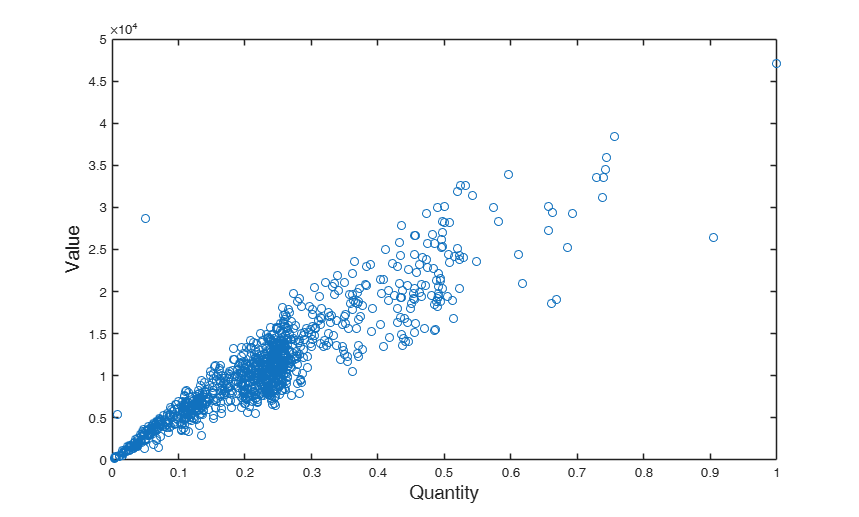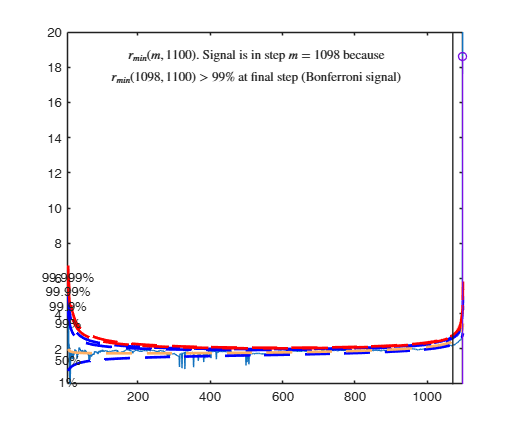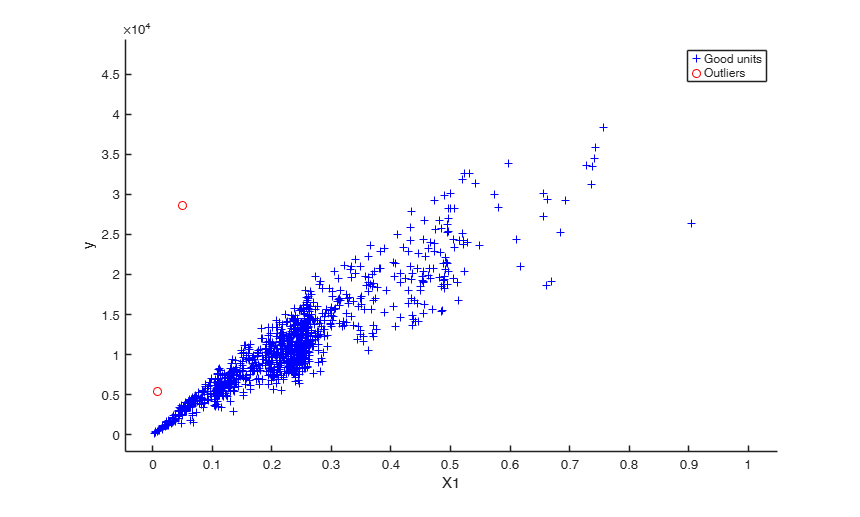FSRH

FSRH gives an automatic outlier detection procedure in heteroskedastic linear regression

Description

out =FSRH(y, X, Z) FSRH with all default options.

out =FSRH(y, X, Z, Name, Value) FSRH with optional arguments.

Examples

expand allFSRH with all default options.

Before running FSRH, data are plotted. Common part to all examples: load tradeH dataset.

y=XX(:,2);
X=XX(:,1);
X=X./max(X);
Z=log(X);
plot(X,y,'o')
fs=14;
ylabel('Value','FontSize',fs)
xlabel('Quantity','FontSize',fs)
out=FSRH(y,X,Z);
Total estimated time to complete LMS:  0.05 seconds
Warning: interchange greater than 10 when m=13
Number of units which entered=14
Warning: interchange greater than 10 when m=353
Number of units which entered=11
Warning: interchange greater than 10 when m=354
Number of units which entered=16
Warning: interchange greater than 10 when m=499
Number of units which entered=21
Warning: interchange greater than 10 when m=502
Number of units which entered=13
Warning: interchange greater than 10 when m=507
Number of units which entered=19
Warning: interchange greater than 10 when m=509
Number of units which entered=11
-------------------------
Signal detection loop
Signal is in penultimate step of the search
rmin(1098,1100)>99.9%
rmin(1098,1100)>99% at final step: Bonferroni signal in the final part of the search.

-------------------
Signal validation exceedance of upper envelopes
Validated signal
Warning: Upper limit of y axis of the mdr forward plot set to 20
-------------------------------
Start resuperimposing envelopes from step m=1097
Superimposition stopped because r_{min}(1098,1099)>99% envelope
$r_{min}(1098,1099)>99$\% envelope
----------------------------
Final output
Number of units declared as outliers=2
Summary of the exceedances
1          99         999        9999       99999
75           6           2           2           2FSRH with optional arguments.

Specifying the search initialization and controlling the y scale in plot.

y=XX(:,2);
X=XX(:,1);
X=X./max(X);
Z=log(X);
out=FSRH(y,X,Z,'init',round(length(y)/2),'plots',1,'ylim',[1.6 3]);

Input Arguments

y — Response variable. Vector.

Response variable, specified as a vector of length n, where n is the number of observations. Each entry in y is the response for the corresponding row of X.

Missing values (NaN's) and infinite values (Inf's) are allowed, since observations (rows) with missing or infinite values will automatically be excluded from the computations.

Data Types: single| double

X — Predictor variables in the regression equation. Matrix.

Matrix of explanatory variables (also called 'regressors') of dimension n x (p-1) where p denotes the number of explanatory variables including the intercept.

Rows of X represent observations, and columns represent variables. By default, there is a constant term in the model, unless you explicitly remove it using input option intercept, so do not include a column of 1s in X. Missing values (NaN's) and infinite values (Inf's) are allowed, since observations (rows) with missing or infinite values will automatically be excluded from the computations.

Data Types: single| double

Z — Predictor variables in the scedastic equation. Matrix.

n x r matrix or vector of length r.

If Z is a n x r matrix it contains the r variables which form the scedastic function as follows (if input option art==1)

$\omega_i = 1 + exp(\gamma_0 + \gamma_1 Z(i,1) + ...+ \gamma_{r} Z(i,r))$ If Z is a vector of length r it contains the indexes of the columns of matrix X which form the scedastic function as follows $\omega_i = 1 + exp(\gamma_0 + \gamma_1 X(i,Z(1)) + ...+ \gamma_{r} X(i,Z(r)))$

Therefore, if for example the explanatory variables responsible for heteroscedasticity are columns 3 and 5 of matrix X, it is possible to use both the sintax:

FSRH(y,X,X(:,[3 5])) or the sintax:

FSRH(y,X,[3 5])

Data Types: single| double

Name-Value Pair Arguments

Specify optional comma-separated pairs of Name,Value arguments. Name is the argument name and Value is the corresponding value. Name must appear inside single quotes (' '). You can specify several name and value pair arguments in any order as Name1,Value1,...,NameN,ValueN.

Example: 'intercept',false , 'modeltype','har' , 'h',round(n*0,75) , 'nsamp',1000 , 'lms',1 , 'plots',1 , 'init',100 starts monitoring from step m=100 , 'tag',{'plmdr' 'plyXplot'}; , 'gridsearch',1 , 'nocheck',true , 'bivarfit',2 , 'multivarfit','1' , 'labeladd','1' , 'nameX',{'NameVar1','NameVar2'} , 'namey','NameOfResponse' , 'bonflev',0.99 , 'msg',1 , 'bsbmfullrank',1

intercept —Indicator for constant term.true (default) | false.

Indicator for the constant term (intercept) in the fit, specified as the comma-separated pair consisting of 'Intercept' and either true to include or false to remove the constant term from the model.

Example: 'intercept',false

Data Types: boolean

modeltype —Parametric function to be used in the skedastic equation.string.

If modeltype is 'arc' (default) than the skedastic function is modelled as follows $\sigma^2_i = \sigma^2 (1 + \exp(\gamma_0 + \gamma_1 Z(i,1) + \cdots + \gamma_{r} Z(i,r)))$ on the other hand, if modeltype is 'har' then traditional formulation due to Harvey is used as follows $\sigma^2_i = \exp(\gamma_0 + \gamma_1 Z(i,1) + \cdots + \gamma_{r} Z(i,r)) =\sigma^2 (\exp(\gamma_1 Z(i,1) + \cdots + \gamma_{r} Z(i,r))$

Example: 'modeltype','har'

Data Types: string

h —The number of observations that have determined the least trimmed squares estimator.scalar.

h is an integer greater or equal than p but smaller then n. Generally if the purpose is outlier detection h=[0.5*(n+p+1)] (default value). h can be smaller than this threshold if the purpose is to find subgroups of homogeneous observations.

Example: 'h',round(n*0,75)

Data Types: double

nsamp —Number of subsamples which will be extracted to find the robust estimator.scalar.

If nsamp=0 all subsets will be extracted.

They will be (n choose p).

Remark: if the number of all possible subset is <1000 the default is to extract all subsets otherwise just 1000.

Example: 'nsamp',1000

Data Types: double

lms —Criterion to use to find the initlal subset to initialize the search.scalar, vector | structure.

lms specifies the criterion to use to find the initlal subset to initialize the search (LMS, LTS with concentration steps, LTS without concentration steps or subset supplied directly by the user).

The default value is 1 (Least Median of Squares is computed to initialize the search). On the other hand, if the user wants to initialze the search with LTS with all the default options for concentration steps then lms=2. If the user wants to use LTS without concentration steps, lms can be a scalar different from 1 or 2. If lms is a struct it is possible to control a series of options for concentration steps (for more details see option lms inside LXS.m) LXS.

If, on the other hand, the user wants to initialize the search with a prespecified set of units there are two possibilities:

1) lms can be a vector with length greater than 1 which contains the list of units forming the initial subset. For example, if the user wants to initialize the search with units 4, 6 and 10 then lms=[4 6 10].

2) lms is a struct which contains a field named bsb which contains the list of units to initialize the search. For example, in the case of simple regression through the origin with just one explanatory variable, if the user wants to initialize the search with unit 3 then lms=struct;

Value Description
bsb

3.

Example: 'lms',1

Data Types: double

plots —Plot on the screen.scalar.

If plots=1 (default) the plot of minimum deletion residual with envelopes based on n observations and the scatterplot matrix with the outliers highlighted is produced.

If plots=2 the user can also monitor the intermediate plots based on envelope superimposition.

else no plot is produced.

Example: 'plots',1

Data Types: double

init —Search initialization.scalar.

It specifies the initial subset size to start monitoring exceedances of minimum deletion residual, if init is not specified it set equal to:

p+1, if the sample size is smaller than 40;

min(3*p+1,floor(0.5*(n+p+1))), otherwise.

Example: 'init',100 starts monitoring from step m=100

Data Types: double

tag —tags to the plots which are created.character | cell array of characters.

This option enables to add a tag to the plots which are created. The default tag names are:

fsr_mdrplot for the plot of mdr based on all the observations;

fsr_yXplot for the plot of y against each column of X with the outliers highlighted;

fsr_resuperplot for the plot of resuperimposed envelopes. The first plot with 4 panel of resuperimposed envelopes has tag fsr_resuperplot1, the second fsr_resuperplot2 ...

If tag is character or a cell of characters of length 1, it is possible to specify the tag for the plot of mdr based on all the observations;

If tag is a cell of length 2 it is possible to control both the tag for the plot of mdr based on all the observations and the tag for the yXplot with outliers highlighted.

If tag is a cell of length 3 the third element specifies the names of the plots of resuperimposed envelopes.

Example: 'tag',{'plmdr' 'plyXplot'};

Data Types: char or cell

gridsearch —Algorithm to be used.scalar.

If gridsearch ==1 grid search will be used else (default) the scoring algorith will be used.

Example: 'gridsearch',1

Data Types: double

nocheck —Check input arguments.boolean.

If nocheck is equal to true no check is performed on matrix y and matrix X. Notice that y and X are left unchanged. In other words the additional column of ones for the intercept is not added. As default nocheck=false.

Example: 'nocheck',true

Data Types: boolean

bivarfit —Superimpose bivariate least square lines.character.

This option adds one or more least square lines, based on SIMPLE REGRESSION of y on Xi, to the plots of y|Xi.

bivarfit = '' is the default: no line is fitted.

bivarfit = '1' fits a single ols line to all points of each bivariate plot in the scatter matrix y|X.

bivarfit = '2' fits two ols lines: one to all points and another to the group of the genuine observations. The group of the potential outliers is not fitted.

bivarfit = '0' fits one ols line to each group. This is useful for the purpose of fitting mixtures of regression lines.

bivarfit = 'i1' or 'i2' or 'i3' etc.

fits an ols line to a specific group, the one with index 'i' equal to 1, 2, 3 etc. Again, useful in case of mixtures.

Example: 'bivarfit',2

Data Types: char

multivarfit —Superimpose multivariate least square lines.character.

This option adds one or more least square lines, based on MULTIVARIATE REGRESSION of y on X, to the plots of y|Xi.

multivarfit = '' is the default: no line is fitted.

multivarfit = '1' fits a single ols line to all points of each bivariate plot in the scatter matrix y|X. The line added to the scatter plot y|Xi is avconst + Ci*Xi, where Ci is the coefficient of Xi in the multivariate regression and avconst is the effect of all the other explanatory variables different from Xi evaluated at their centroid (that is overline{y}'C)) multivarfit = '2' equal to multivarfit ='1' but this time we also add the line based on the group of unselected observations (i.e. the normal units).

Example: 'multivarfit','1'

Data Types: char

If this option is '1', we label the outliers with the unit row index in matrices X and y. The default value is labeladd='', i.e. no label is added.

Data Types: char

nameX —Add variable labels in plot.cell array of strings.

labels of the variables of the regression dataset. If it is empty (default) the sequence X1, ..., Xp will be created automatically

Example: 'nameX',{'NameVar1','NameVar2'}

Data Types: cell

label of the response

Example: 'namey','NameOfResponse'

Data Types: char

ylim —Control y scale in plot.vector.

minimum and maximum on the y axis. Default value is '' (automatic scale)

Example: 'ylim','[0,10]' sets the minim value to 0 and the max to 10 on the y axis

Data Types: double

xlim —Control x scale in plot.vector.

minimum and maximum on the x axis. Default value is '' (automatic scale)

Example: 'xlim','[0,10]' sets the minim value to 0 and the max to 10 on the x axis

Data Types: double

bonflev —Signal to use to identify outliers.scalar.

option to be used if the distribution of the data is strongly non normal and, thus, the general signal detection rule based on consecutive exceedances cannot be used. In this case bonflev can be:

- a scalar smaller than 1 which specifies the confidence level for a signal and a stopping rule based on the comparison of the minimum MD with a Bonferroni bound. For example if bonflev=0.99 the procedure stops when the trajectory exceeds for the first time the 99% bonferroni bound.

- A scalar value greater than 1. In this case the procedure stops when the residual trajectory exceeds for the first time this value.

Default value is '', which means to rely on general rules based on consecutive exceedances.

Example: 'bonflev',0.99

Data Types: double

msg —Level of output to display.scalar.

It controls whether to display or not messages on the screen If msg==1 (default) messages are displayed on the screen about step in which signal took place else no message is displayed on the screen

Example: 'msg',1

Data Types: double

bsbmfullrank —Dealing with singluar X matrix.scalar.

It tells how to behave in case subset at step m (say bsbm) produces a non singular X. In other words, this options controls what to do when rank(X(bsbm,:)) is smaller then number of explanatory variables. If bsbmfullrank =1 (default) these units (whose number is say mnofullrank) are constrained to enter the search in the final n-mnofullrank steps else the search continues using as estimate of beta at step m the estimate of beta found in the previous step.

Example: 'bsbmfullrank',1

Data Types: double

Output Arguments

out — description Structure

T consists of a structure 'out' containing the following fields:

Value Description
ListOut

k x 1 vector containing the list of the units declared as outliers or NaN if the sample is homogeneous. This field in future releases will be deleted bacause it will be replaced by out.outliers.

outliers

k x 1 vector containing the list of the units declared as outliers or NaN if the sample is homogeneous.

beta

p-by-1 vector containing the estimated regression parameters (in step n-k).

hetero

r-by-1 vector containing the estimated parameters of the scedastic parameters (in step n-k)

scale

scalar containing the estimate of the scale (sigma).

mdr

(n-init) x 2 matrix:

1st col = fwd search index;

2nd col = value of minimum deletion residual in each step of the fwd search.

Un

(n-init) x 11 Matrix which contains the unit(s) included in the subset at each step of the fwd search.

REMARK: in every step the new subset is compared with the old subset. Un contains the unit(s) present in the new subset but not in the old one.

Un(1,2) for example contains the unit included in step init+1.

Un(end,2) contains the units included in the final step of the search.

nout

2 x 5 matrix containing the number of times mdr went out of particular quantiles.

First row contains quantiles 1 99 99.9 99.99 99.999.

Second row contains the frequency distribution.

constr

This output is produced only if the search found at a certain step a non singular matrix X. In this case the search run in a constrained mode, that is including the units which produced a singular matrix in the last n-constr steps. out.constr is a vector which contains the list of units which produced a singular X matrix

class

'FSRH'.

Atkinson, A.C., Riani, M. and Torti, F. (2016), Robust methods for heteroskedastic regression, "Computational Statistics and Data Analysis", Vol. 104, pp. 209-222, http://dx.doi.org/10.1016/j.csda.2016.07.002 [ART]